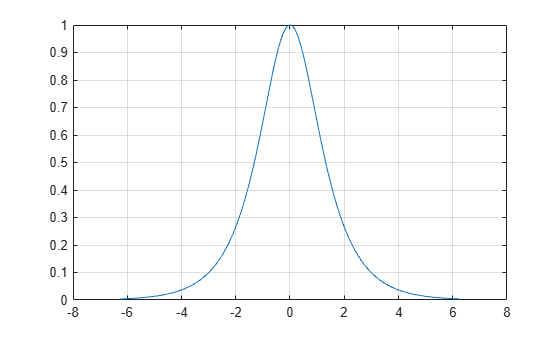# sech

Hyperbolic secant

## Syntax

``Y = sech(X)``

## Description

example

````Y = sech(X)` returns the hyperbolic secant of the elements of `X`. The `sech` function operates element-wise on arrays. The function accepts both real and complex inputs. All angles are in radians.```

## Examples

collapse all

Create a vector and calculate the hyperbolic secant of each value.

```X = [0 pi 2*pi 3*pi]; Y = sech(X)```
```Y = 1×4 1.0000 0.0863 0.0037 0.0002 ```

Plot the hyperbolic secant over the domain $-2\pi \le x\le 2\pi$.

```x = -2*pi:0.01:2*pi; y = sech(x); plot(x,y) grid on```## Input Arguments

collapse all

Input angles in radians, specified as a scalar, vector, matrix, or multidimensional array.

Data Types: `single` | `double`
Complex Number Support: Yes

collapse all

### Hyperbolic Secant

The hyperbolic secant of x is equal to the inverse of the hyperbolic cosine

`$\text{sech}\left(x\right)=\frac{1}{\mathrm{cosh}\left(x\right)}=\frac{2}{{e}^{x}+{e}^{-x}}.$`

In terms of the traditional secant function with a complex argument, the identity is

`$\text{sech}\left(x\right)=\mathrm{sec}\left(ix\right)\text{\hspace{0.17em}}.$`

## Version History

Introduced before R2006a